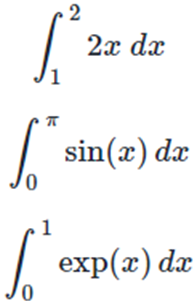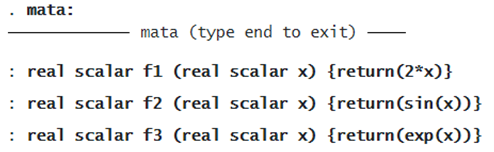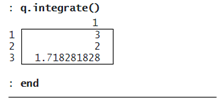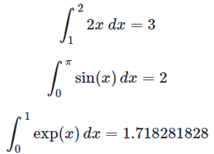VECTORIZED NUMERICAL INTEGRATION

VECTORIZED NUMERICAL INTEGRATION IN ACTION

The new Mata class QuadratureVec() is created for the storage and computation of vectorized numerical integration.

Here is an example to approximate the following three integrals:We define evaluator functions f1()f2(), and f3():Having defined the evaluator functions, we follow the four steps that are required each time we use the QuadratureVec() class. First, we create an instance q of the QuadratureVec() class:

```: q = QuadratureVec()
```

Second, we use setEvaluator() to point to the evaluator functions, defined as the column vector evaluator:

```: evaluator = (&f1() \ &f2() \ &f3())
: q.setEvaluator(evaluator)
```

Third, we use setLimits() to specify the lower and upper limits, defined as limits:

```: limits = ((1, 2) \ (0,pi()) \ (0,1))
: q.setLimits(limits)
```

Fourth, we use integrate() to compute the approximations:We find that